• 树形结构
• 二叉树
• 完全二叉树和满二叉树
• 数组

# Part 1：啥是堆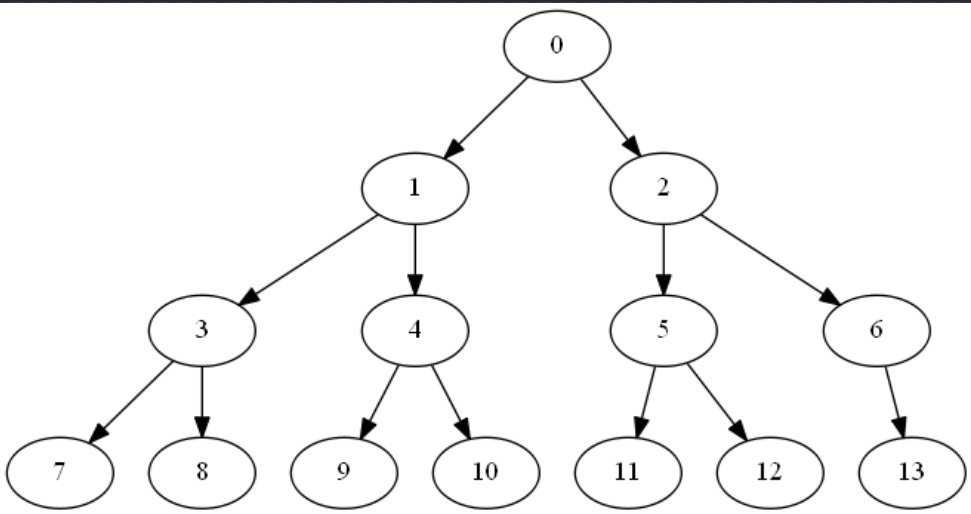$Father = \lfloor \frac{child1 + child2}{2} \rfloor$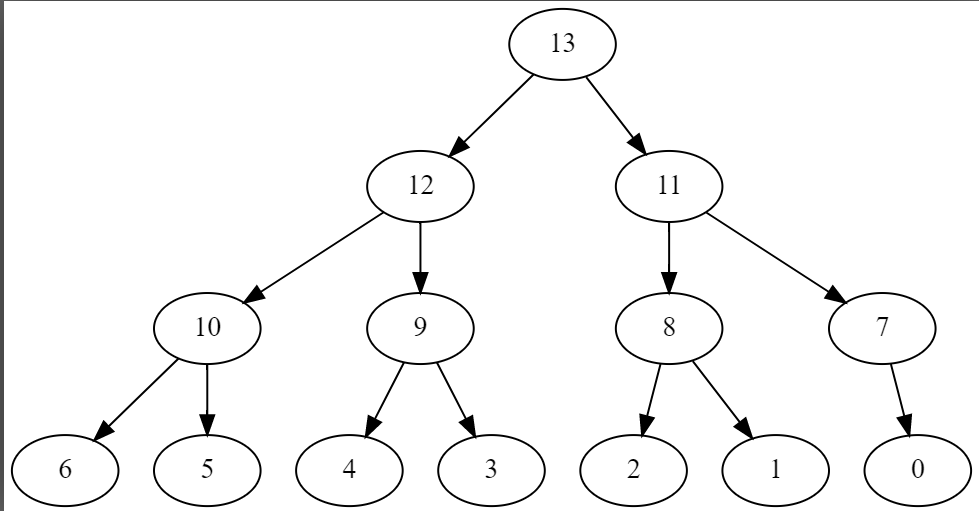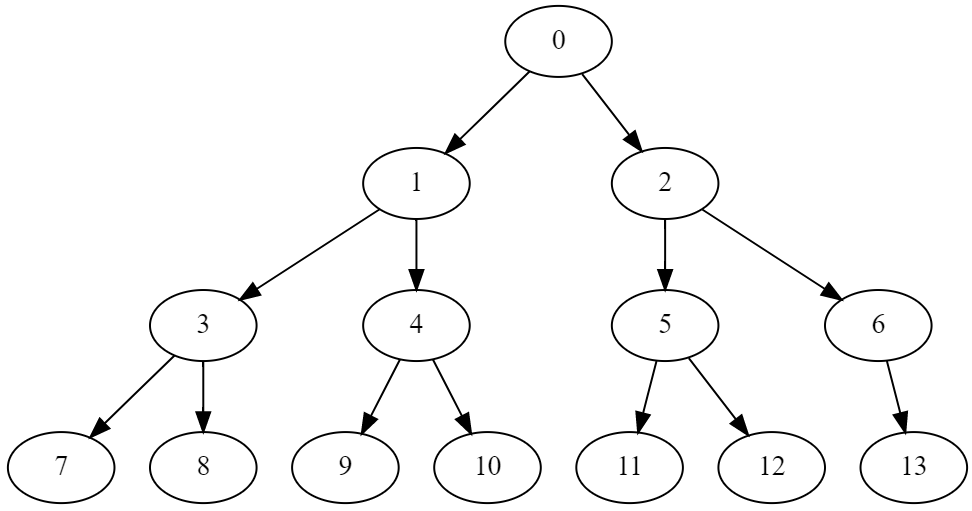# Part 2：堆的基本操作

• 上浮（上调节点） shift_up
• 下沉（下调节点） shift_down
• 插入（插入节点） push
• 弹出（弹出节点）pop
• 取顶（得到最顶端的节点） top
• 堆排序（排序）heap_sort

## 上浮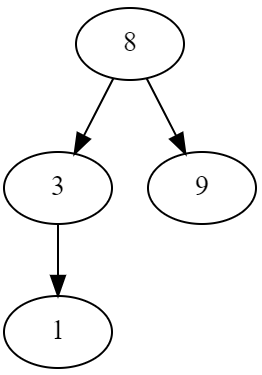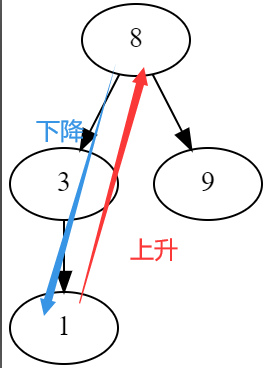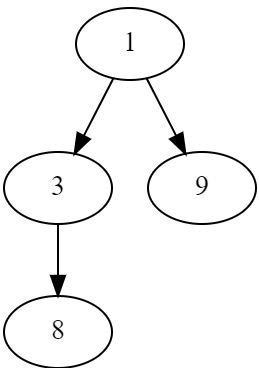//这里的heap数组是存放堆的数组
//请注意：不是所有的堆一开始就是小根堆和大根堆
//在进行几次上浮和下沉后，才能保证他是一个大根堆或者小根堆
void Shift_up(int i){
while(i/2 >= 1){ //一直比较到最顶端
if(heap[i] < heap[i/2]){ //int的特性：int/int = floor(double/double)
swap(heap[i],heap[i/2]);
i /= 2; //这个时候相当于指向i的一个指针要向上走，走到他的父节点
}
else break;
}


## 下沉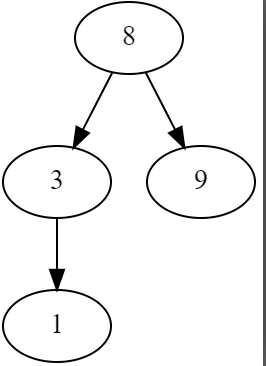void Shift_down(int i ,int n){//n表示当前有n个节点
while(i*2 <= n){
int t = i * 2 ;
if(t+1 <= n && heap[T+1] < heap[T]) T++;
if(heap[i] < heap[T]){
swap(heap[ i ],heap[T] );
i = T;
}
else break;
}


## 插入

void Push(x){
n++; //n是表示当前有多少个元素
heap[n] = x;
Shift_up(n);
}


## 弹出

void Pop(x){
swap(heap,heap[n]);
n--;
Shift_down( 1 );
}


## 取顶

int top(){
return heap;
}


## 堆排序

void Heap_sort(int arr[]){
k=0;
while(size > 0){
k++;
arr[k] = top();
pop();
}
}


# Part 3：优先级队列

$n$个小朋友排队打饭，他们所需要的时间分别为$n_i$，当前一个小朋友打饭的时候，后面的小朋友就要等。怎么排序才能让等待时间之和最短。

（以下是待更新部分）

# Part 6.4：斜堆

posted @ 2020-03-22 14:03  SD！LTF  阅读(...)  评论(...编辑  收藏